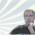Wednesday, November 18, 2015

Searching With Text Boxes In Page

Searching  With Text Boxes In Worksheet

The template has got 56.666 rows.That is pretty big a page.

But don't worry. Searching and filtering can be done easily with  text boxes in the page.Besides can be filtering using multiple criteria inputs from text boxes.
You can use this example for your own template.

Example code -for textbox1-  :

Private Sub TextBox1_Change()
On Error Resume Next
metin = TextBox1.Value
Set bul = Range("j4:j65536").Find(What:=metin)
Selection.AutoFilter field:=10, Criteria1:=TextBox1.Value & "*"
If metin = "" Then
Selection.AutoFilter
[j4].Activate
End If
End Sub

1.Dear,

If i use your excel sample code and try to search there is no records.

The functions are working good, but no valid data.

2.I have used this:

'For More : merkez-ihayat.blogspot.com
Dim metin As String

Private Sub CommandButton1_Click()
TextBox1.Text = ""
TextBox2.Text = ""
TextBox3.Text = ""

End Sub

Private Sub TextBox1_Change()
On Error Resume Next
metin = TextBox1.Value
Set bul = Range("a5:a65536").Find(What:=metin)
Selection.AutoFilter field:=5, Criteria1:=TextBox1.Value & "*"
If metin = "" Then
Selection.AutoFilter
[a5].Activate
End If
End Sub

Private Sub TextBox2_Change()
On Error Resume Next
metin = TextBox2.Value
Set bul = Range("c5:c65536").Find(What:=metin)
Selection.AutoFilter field:=9, Criteria1:=TextBox2.Value & "*"
If metin = "" Then
Selection.AutoFilter
[c5].Activate
End If
End Sub
Private Sub TextBox3_Change()
On Error Resume Next
metin = TextBox3.Value
Set bul = Range("b5:b65536").Find(What:=metin)
Selection.AutoFilter field:=6, Criteria1:=TextBox3.Value & "*"
If metin = "" Then
Selection.AutoFilter
[b5].Activate
End If
End Sub

1.Are the column numbers right? For example :
"field:=5" , it refers to column 5.

3.yes, i have done. But still doesn't work.
I can send you my sample file.

4.Hi there. It went well until on numeric parts. It can only find alphabet & alpha-numeric. But not solid digits. Pls help.